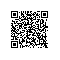# 《R的极客理想——高级开发篇 A》一一1.4　常用连续型分布介绍及R语言实现

### 1.4　常用连续型分布介绍及R语言实现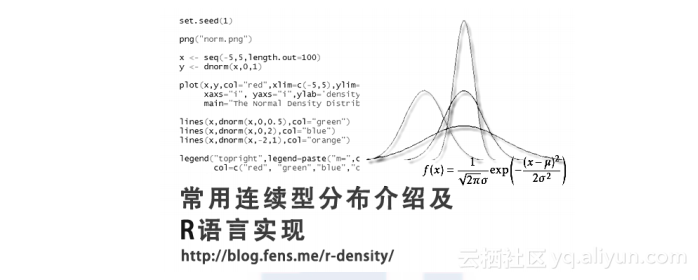1.4.1　均匀分布

Windows 7 64bit
R: 3.1.1 x86_64-w64-mingw32/x64 (64-bit)

1.?概率密度函数（1.13）

set.seed(1) # 设置随机种子
x<-seq(0,10,length.out=1000) # 取从0～10顺序取的1000个点
y<-dunif(x,0,1) # 计算服从均匀分布U(0,1)，1000个点的概率密度函数的值

plot(x,y,col="red",xlim=c(0,10),ylim=c(0,1.2),type='l',xaxs="i", yaxs="i",

ylab='density',xlab='',main="The Uniform Density Distribution")
# 画出概率密度函数曲线，如图1-12所示

lines(x,dnorm(x,0,0.5),col="green") # 计算服从均匀分布U(0,0.5)的值，并增加一条曲线
lines(x,dnorm(x,0,2),col="blue") # 计算服从均匀分布U(0,2)的值，并增加一条曲线
lines(x,dnorm(x,-2,1),col="orange") # 计算服从均匀分布U(-2，1)的值，并增加一条曲线
lines(x,dnorm(x,4,2),col="purple") # 计算服从均匀分布U(4，2)的值，并增加一条曲线
legend("topright",legend=paste("m=",c(0,0,0,-2,4)," sd=", c(1,0.5,2,1,2)),

lwd=1, col=c("red", "green","blue","orange","purple"))  # 在右上角增加图例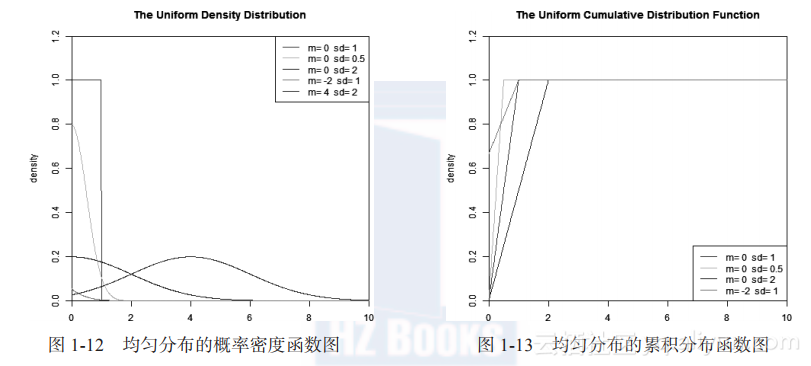2.?累积分布函数set.seed(1) # 设置随机种子
x<-seq(0,10,length.out=1000) # 取从0～10顺序取的1000个点
y<-punif(x,0,1) # 计算服从均匀分布U(0,1)，1000个点的累积分布函数的值

plot(x,y,col="red",xlim=c(0,10),ylim=c(0,1.2),type='l',xaxs="i", yaxs="i",

> lines(x,punif(x,0,0.5),col="green")
> lines(x,punif(x,0,2),col="blue")
> lines(x,punif(x,-2,1),col="orange")
> legend("bottomright",legend=paste("m=",c(0,0,0,-2)," sd=", c(1,0.5,2,1)),
lwd=1, col=c("red", "green","blue","orange","purple"))


3.?分布检验
Kolmogorov-Smirnov连续分布检验是检验单一样本是不是服从某一预先假设的特定分布的方法。把样本数据的累计频数分布与特定理论分布相比较，若两者间的差距很小，则推论该样本取自某特定分布族。

D=max|F0(x)-Fn(x)|
D值越小，越接近0，表示样本数据越接近均匀分布
p值，如果p值小于显著性水平α(0.05)，则拒绝H0

set.seed(1) # 设置随机种子
S<-runif(1000) # 生成服从均匀分布的1000点
ks.test(S, "punif") # Kolmogorov-Smirnov检验

One-sample Kolmogorov-Smirnov test

data: S

D = 0.0244, p-value = 0.5928
alternative hypothesis: two-sided
D值很小，p值>0.05，不能拒绝原假设，所以数据集S符合均匀分布！

1.4.2　正态分布

1.?概率密度函数

![image](https://yqfile.alicdn.com/d771e1d54955ebba72de95599ae983e435b5ac0a.png)

（1.15）

set.seed(1)
x <- seq(-5,5,length.out=100) # 取从-5到5的100个点
y <- dnorm(x,0,1) # 计算服从正态分布N(0,1)，100个点的概率密度函数的值

plot(x,y,col="red",xlim=c(-5,5),ylim=c(0,1),type='l',xaxs="i", yaxs="i",ylab

='density',xlab='',main="The Normal Density Distribution")
# 画出概率密度函数曲线，如图1-14所示

lines(x,dnorm(x,0,0.5),col="green")
lines(x,dnorm(x,0,2),col="blue")
lines(x,dnorm(x,-2,1),col="orange")
legend("topright",legend=paste("m=",c(0,0,0,-2)," sd=", c(1,0.5,2,1)), lwd=1,

  col=c("red", "green","blue","orange"))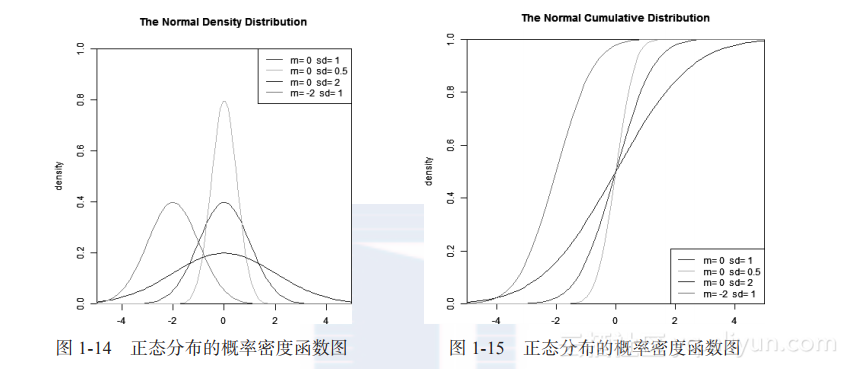2.?累积分布函数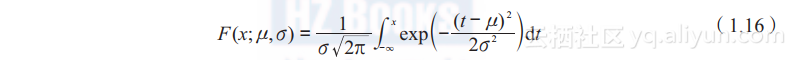set.seed(1)
x <- seq(-5,5,length.out=100)
y <- pnorm(x,0,1) # 计算服从正态分布N(0,1)，100个点的累积分布函数的值

> plot(x,y,col="red",xlim=c(-5,5),ylim=c(0,1),type='l',xaxs="i", yaxs="i",
ylab='F(x)',xlab='',main="The Normal Cumulative Distribution")
# 画出累积分布函数曲线，如图1-15所示
> lines(x,pnorm(x,0,0.5),col="green")
> lines(x,pnorm(x,0,2),col="blue")
> lines(x,pnorm(x,-2,1),col="orange")
> legend("bottomright",legend=paste("m=",c(0,0,0,-2)," sd=", c(1,0.5,2,1)),
lwd=1,col=c("red", "green","blue","orange"))


3.?分布检验
Shapiro-Wilk正态分布检验用来检验数据是否符合正态分布，同线性回归的方法一样，是检验其与回归曲线的残差。该方法推荐在样本量很小的时候使用，一般是样本在3～5000之间。
Shapiro-Wilk正态分布检验的原假设为H0:数据集符合正态分布，统计量W为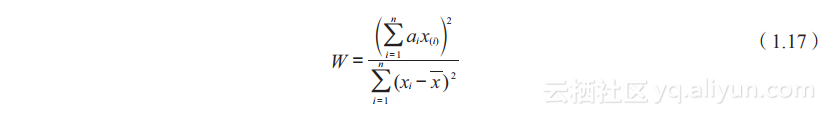（1.17）

set.seed(1)
S<-rnorm(1000) # 生成服从正态分布的1000点
shapiro.test(S) # Shapiro-Wilk正态分布检验

Shapiro-Wilk normality test

data: S

W = 0.9988, p-value = 0.7256

set.seed(1)
S<-rnorm(1000)
ks.test(S, "pnorm") # Kolmogorov-Smirnov检验

One-sample Kolmogorov-Smirnov test

data: S

D = 0.0211, p-value = 0.7673
alternative hypothesis: two-sided

1.4.3　指数分布

1.?概率密度函数

![image](https://yqfile.alicdn.com/082aa28873222b8cfd4bf8ff26da38c9b8d4e307.png)

（1.18）

set.seed(1)
x<-seq(-1,2,length.out=100)
y<-dexp(x,0.5) # 计算服从指数分布e(0.5)，100个点的概率密度函数的值

plot(x,y,col="red",xlim=c(0,2),ylim=c(0,5),type='l',xaxs="i", yaxs="i",ylab=

'density',xlab='',main="The Exponential Density Distribution")
# 画出概率密度函数曲线，如图1-16所示

lines(x,dexp(x,1),col="green")
lines(x,dexp(x,2),col="blue")
lines(x,dexp(x,5),col="orange")
legend("topright",legend=paste("rate=",c(.5, 1, 2,5)), lwd=1,col=c("red",

"green","blue","orange"))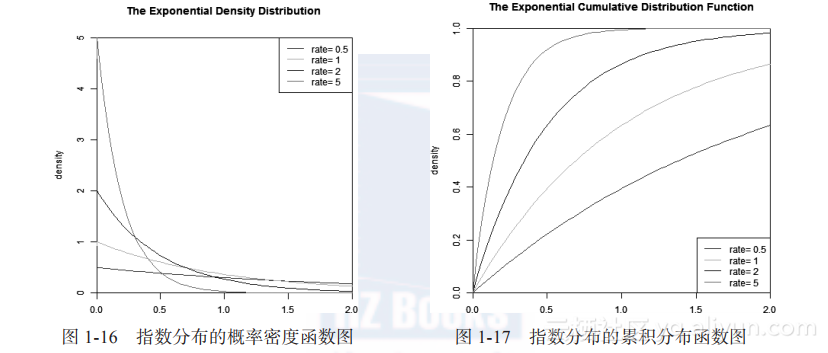2.?累积分布函数set.seed(1)
x<-seq(-1,2,length.out=100)
y<-pexp(x,0.5) # 计算服从指数分布e(0.5)，100个点的累积分布函数的值

plot(x,y,col="red",xlim=c(0,2),ylim=c(0,1),type='l',xaxs="i", yaxs="i",ylab=

'F(x)',xlab='',main="The Exponential Cumulative Distribution Function")
# 画出累积分布函数曲线，如图1-17所示

lines(x,pexp(x,1),col="green")
lines(x,pexp(x,2),col="blue")
lines(x,pexp(x,5),col="orange")
legend("bottomright",legend=paste("rate=",c(.5, 1, 2,5)), lwd=1, col=c("red",

"green","blue","orange"))

3.?分布检验

set.seed(1)
S<-rexp(1000)
ks.test(S, "pexp") # Kolmogorov-Smirnov检验

One-sample Kolmogorov-Smirnov test

data: S

D = 0.0387, p-value = 0.1001
alternative hypothesis: two-sided

1.4.4　伽玛分布

![image](https://yqfile.alicdn.com/9b90ed301ecd0deb1ca44aadc517357373c4ba14.png)

（1.20）
1.?概率密度函数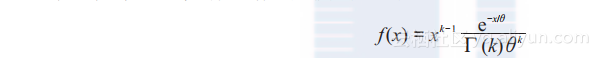（1.21）

set.seed(1)
x<-seq(0,10,length.out=100)
y<-dgamma(x,1,2) # 计算服从伽玛分布Ga(1,2)，100个点的概率密度函数的值

plot(x,y,col="red",xlim=c(0,10),ylim=c(0,2),type='l',xaxs="i", yaxs="i",ylab=

'density',xlab='',main="The Gamma Density Distribution")
# 画出概率密度函数曲线，如图1-18所示


lines(x,dgamma(x,2,2),col="green")
lines(x,dgamma(x,3,2),col="blue")
lines(x,dgamma(x,5,1),col="orange")
lines(x,dgamma(x,9,1),col="black")
legend("topright",legend=paste("shape=",c(1,2,3,5,9)," rate=", c(2,2,2,1,1)),

lwd=1, col=c("red", "green","blue","orange","black"))

2.?累积分布函数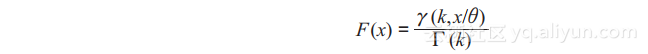（1.22）

set.seed(1)
x<-seq(0,10,length.out=100)
y<-pgamma(x,1,2) # 计算服从伽玛分布Ga(1,2)，100个点的累积分布函数的值

plot(x,y,col="red",xlim=c(0,10),ylim=c(0,1),type='l',xaxs="i", yaxs="i",ylab=

'F(x)',xlab='',main="The Gamma Cumulative Distribution Function")
# 画出累积分布函数曲线，如图1-19所示

lines(x,pgamma(x,2,2),col="green")
lines(x,pgamma(x,3,2),col="blue")
lines(x,pgamma(x,5,1),col="orange")
lines(x,pgamma(x,9,1),col="black")
legend("bottomright",legend=paste("shape=",c(1,2,3,5,9)," rate=",

c(2,2,2,1,1)), lwd=1, col=c("red", "green","blue","orange","black"))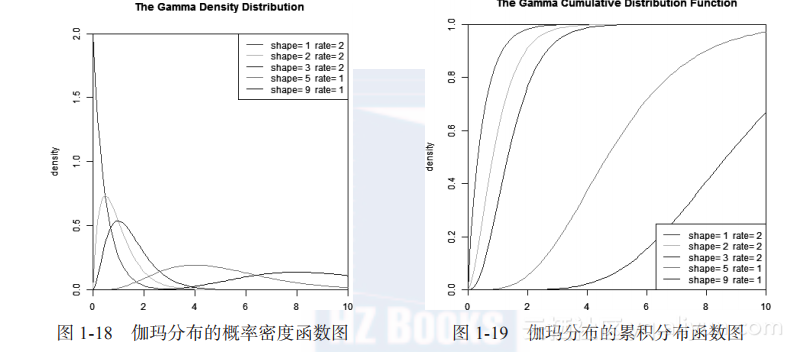3.?分布检验

set.seed(1)
S<-rgamma(1000,1)
ks.test(S, "pgamma", 1) # Kolmogorov-Smirnov检验

One-sample Kolmogorov-Smirnov test

data: S

D = 0.0363, p-value = 0.1438
alternative hypothesis: two-sided

ks.test(S, "pgamma", 2) # Kolmogorov-Smirnov检验

One-sample Kolmogorov-Smirnov test

data: S

D = 0.3801, p-value < 2.2e-16
alternative hypothesis: two-sided

1.4.5　韦布尔分布

1.?概率密度函数（1.23）

set.seed(1)
x<- seq(0, 2.5, length.out=1000)
y<- dweibull(x, 0.5) # 计算服从韦布尔分布W(0.5,1)，1000个点的概率密度函数的值

plot(x, y, type="l", col="blue",xlim=c(0, 2.5),ylim=c(0, 6),xaxs="i", yaxs=

"i",ylab='density',xlab='',main="The Weibull Density Distribution")
# 画出概率密度函数曲线，如图1-20所示

lines(x, dweibull(x, 1), type="l", col="red")
lines(x, dweibull(x, 1.5), type="l", col="magenta")
lines(x, dweibull(x, 5), type="l", col="green")
lines(x, dweibull(x, 15), type="l", col="purple")
legend("topright", legend=paste("shape =", c(.5, 1, 1.5, 5, 15)),

lwd=1,col=c("blue", "red", "magenta", "green","purple"))

2.?累积分布函数

![image](https://yqfile.alicdn.com/5d1088610a4e460282355fbac62ac45008ed7dec.png)

（1.24）

set.seed(1)
x<- seq(0, 2.5, length.out=1000)
y<- pweibull(x, 0.5) # 计算服从weibull分布W(0.5,1)，1000个点的累积分布函数的值

plot(x, y, type="l", col="blue",xlim=c(0, 2.5),ylim=c(0, 1.2),xaxs="i", yaxs=

"i",ylab='F(x)',xlab='',main="The Weibull Cumulative Distribution Function")
# 画出累积分布函数曲线，如图1-21所示

lines(x, pweibull(x, 1), type="l", col="red")
lines(x, pweibull(x, 1.5), type="l", col="magenta")
lines(x, pweibull(x, 5), type="l", col="green")
lines(x, pweibull(x, 15), type="l", col="purple")
legend("bottomright", legend=paste("shape =", c(.5, 1, 1.5, 5, 15)), lwd=1,

col=c("blue", "red", "magenta", "green","purple"))

图1-20　韦布尔分布的概率密度函数图　 　 图1-21　韦布尔分布的累积分布函数图
3.?分布检验

set.seed(1)
S<-rweibull(1000,1)
ks.test(S, "pweibull",1) # Kolmogorov-Smirnov检验

One-sample Kolmogorov-Smirnov test

data: S

D = 0.0244, p-value = 0.5928
alternative hypothesis: two-sided

1.4.6　卡方分布

1.?概率密度函数

![image](https://yqfile.alicdn.com/22c873c2739421fd52f0651925feeb8976fb7fe6.png)

（1.25）

set.seed(1)
x<-seq(0,10,length.out=1000)
y<-dchisq(x,1) # 计算服从卡方分布X(1)，1000个点的概率密度函数的值

plot(x,y,col="red",xlim=c(0,5),ylim=c(0,2),type='l',xaxs="i", yaxs="i",ylab=

'density',xlab='',main="The Chisq Density Distribution")
# 画出概率密度函数曲线，如图1-22所示

lines(x,dchisq(x,2),col="green")
lines(x,dchisq(x,3),col="blue")
lines(x,dchisq(x,10),col="orange")
legend("topright",legend=paste("df=",c(1,2,3,10)), lwd=1, col=c("red",

"green","blue","orange"))

2.?累积分布函数（1.26）

set.seed(1)
x<-seq(0,10,length.out=1000)
y<-pchisq(x,1) # 计算服从卡方分布X(1)，1000个点的累积分布函数的值

plot(x,y,col="red",xlim=c(0,10),ylim=c(0,1),type='l',xaxs="i", yaxs="i",ylab=

'F(x)',xlab='',main="The Chisq Cumulative Distribution Function")
# 画出累积分布函数曲线，如图1-23所示

lines(x,pchisq(x,2),col="green")
lines(x,pchisq(x,3),col="blue")
lines(x,pchisq(x,10),col="orange")
legend("topleft",legend=paste("df=",c(1,2,3,10)), lwd=1, col=c("red", "green",

"blue","orange"))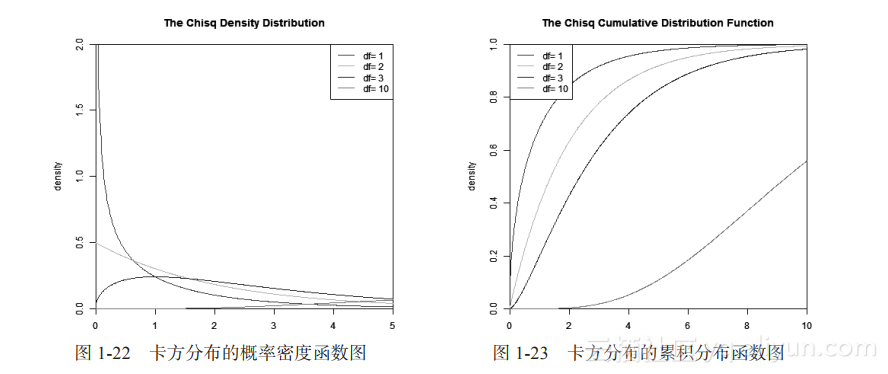3.?分布检验

set.seed(1)
S<-rchisq(1000,1)
ks.test(S, "pchisq",1) # Kolmogorov-Smirnov检验

One-sample Kolmogorov-Smirnov test

data: S

D = 0.0254, p-value = 0.5385
alternative hypothesis: two-sided

1.4.7　F分布
F分布是一种连续型概率分布，广泛应用于似然比率检验，特别是ANOVA中。F分布的定义为：设X，Y为两个独立的随机变量，X服从自由度为d1的卡方分布，Y服从自由度为d2的卡方分布，则 服从自由度为d1和d2的F分布。
F分布是一种非对称分布，它有两个自由度，即d1和d2，相应的分布记为F(d1, d2），d1通常称为分子自由度，d2通常称为分母自由度。F分布是一个以自由度d1和d2为参数的分布族，不同的自由度决定了F分布的形状。
1.?概率密度函数
F分布的概率密度函数公式为（1.27）：

![image](https://yqfile.alicdn.com/1ab844fcb88b9c4055474d0232f1e7a643124e0d.png)

（1.27）

set.seed(1)
x<-seq(0,5,length.out=1000)
y<-df(x,1,1,0) # 计算服从F分布F(1,1,0)，1000个点的概率密度函数的值

plot(x,y,col="red",xlim=c(0,5),ylim=c(0,1),type='l',xaxs="i", yaxs="i",ylab=

'density',xlab='',main="The F Density Distribution")
# 画出概率密度函数曲线，如图1-24所示

lines(x,df(x,1,1,2),col="green")
lines(x,df(x,2,2,2),col="blue")
lines(x,df(x,2,4,4),col="orange")
legend("topright",legend=paste("df1=",c(1,1,2,2),"df2=",c(1,1,2,4)," ncp=",

c(0,2,2,4)), lwd=1, col=c("red", "green","blue","orange"))

2.?累积分布函数

F分布的累积分布函数公式为（1.28）：

![image](https://yqfile.alicdn.com/b184620e939fa865a8548eefb6a943db9140d9e6.png)

（1.28）

set.seed(1)
x<-seq(0,5,length.out=1000)
y<-df(x,1,1,0) # 计算服从F分布F(1,1,0)，1000个点的累积分布函数的值

plot(x,y,col="red",xlim=c(0,5),ylim=c(0,1),type='l',xaxs="i", yaxs="i",ylab=

'F(x)',xlab='',main="The F Cumulative Distribution Function")
# 画出累积分布函数曲线，如图1-25所示

lines(x,pf(x,1,1,2),col="green")
lines(x,pf(x,2,2,2),col="blue")
lines(x,pf(x,2,4,4),col="orange")
legend("topright",legend=paste("df1=",c(1,1,2,2),"df2=",c(1,1,2,4)," ncp=",

c(0,2,2,4)), lwd=1, col=c("red", "green","blue","orange"))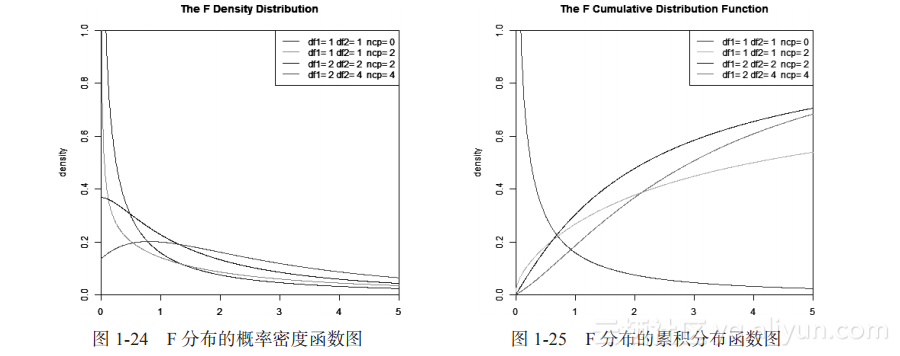3.?分布检验

set.seed(1)
S<-rf(1000,1,1,2)
ks.test(S, "pf", 1,1,2) # Kolmogorov-Smirnov检验

One-sample Kolmogorov-Smirnov test

data: S

D = 0.0113, p-value = 0.9996
alternative hypothesis: two-sided

1.4.8　t分布

1.?概率密度函数
t分布的概率密度函数公式为（1.29）

set.seed(1)
x<-seq(-5,5,length.out=1000)
y<-dt(x,1,0) # 计算服从t分布T(1,0)，1000个点的概率密度函数的值

plot(x,y,col="red",xlim=c(-5,5),ylim=c(0,0.5),type='l',xaxs="i", yaxs="i",ylab

='density',xlab='',main="The T Density Distribution")
# 画出概率密度函数曲线，如图1-26所示

lines(x,dt(x,5,0),col="green")
lines(x,dt(x,5,2),col="blue")
lines(x,dt(x,50,4),col="orange")
legend("topleft",legend=paste("df=",c(1,5,5,50)," ncp=", c(0,0,2,4)), lwd=1,

col=c("red", "green","blue","orange"))

2.?累积分布函数

t分布的累积分布函数公式为（1.30）：

![image](https://yqfile.alicdn.com/09a7278f12b38f736c9d310f596b2af93e5a5118.png)

（1.30）

set.seed(1)
x<-seq(-5,5,length.out=1000)
y<-pt(x,1,0) # 计算服从T分布T(1,0)，1000个点的累积分布函数的值

plot(x,y,col="red",xlim=c(-5,5),ylim=c(0,0.5),type='l',xaxs="i", yaxs="i",ylab

='F(x)',xlab='',main="The T Cumulative Distribution Function")
# 画出累积分布函数曲线，如图1-27所示

lines(x,pt(x,5,0),col="green")
lines(x,pt(x,5,2),col="blue")
lines(x,pt(x,50,4),col="orange")
legend("topleft",legend=paste("df=",c(1,5,5,50)," ncp=", c(0,0,2,4)), lwd=1,

col=c("red", "green","blue","orange"))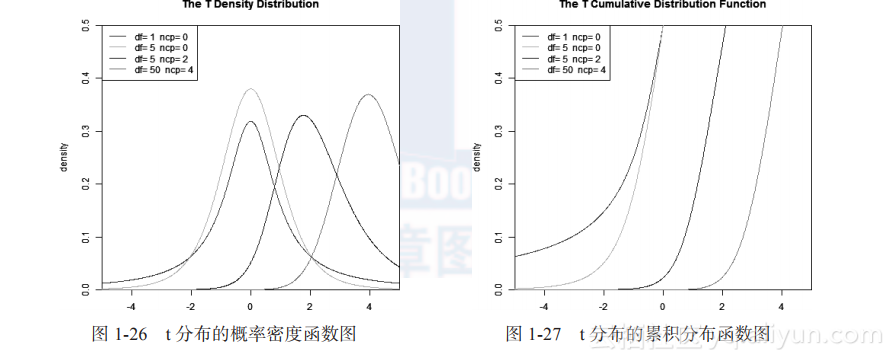3.?分布检验

set.seed(1)
S<-rt(1000, 1,2)
ks.test(S, "pt", 1, 2) # Kolmogorov-Smirnov检验

One-sample Kolmogorov-Smirnov test

data: S

D = 0.0253, p-value = 0.5461
alternative hypothesis: two-sided

1.4.9　贝塔分布

1.?概率密度函数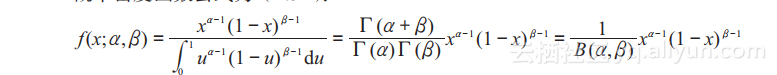（1.31）

set.seed(1)
x<-seq(-5,5,length.out=10000)
y<-dbeta(x,0.5,0.5) # 计算服从贝塔分布B(0.5,0.5)，10000个点的概率密度函数的值

plot(x,y,col="red",xlim=c(0,1),ylim=c(0,6),type='l',xaxs="i", yaxs="i",ylab=

'density',xlab='',main="The Beta Density Distribution")
# 画出概率密度函数曲线，如图1-28所示

lines(x,dbeta(x,5,1),col="green")
lines(x,dbeta(x,1,3),col="blue")
lines(x,dbeta(x,2,2),col="orange")
lines(x,dbeta(x,2,5),col="black")
legend("top",legend=paste("a=",c(.5,5,1,2,2)," b=", c(.5,1,3,2,5)), lwd=1,

col=c("red", "green","blue","orange","black"))

2.?累积分布函数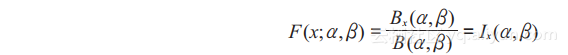（1.32）

set.seed(1)
x<-seq(-5,5,length.out=10000)
y<-pbeta(x,0.5,0.5) # 计算服从贝塔分布B(0.5,0.5)，10000个点的累积分布函数的值

plot(x,y,col="red",xlim=c(0,1),ylim=c(0,1),type='l',xaxs="i", yaxs="i",ylab=

'F(x)',xlab='',main="The Beta Cumulative Distribution Function")
# 画出累积分布函数曲线，如图1-29所示

lines(x,pbeta(x,5,1),col="green")
lines(x,pbeta(x,1,3),col="blue")
lines(x,pbeta(x,2,2),col="orange")
lines(x,pbeta(x,2,5),col="black")
legend("topleft",legend=paste("a=",c(.5,5,1,2,2)," b=", c(.5,1,3,2,5)), lwd=1,

col=c("red", "green","blue","orange","black"))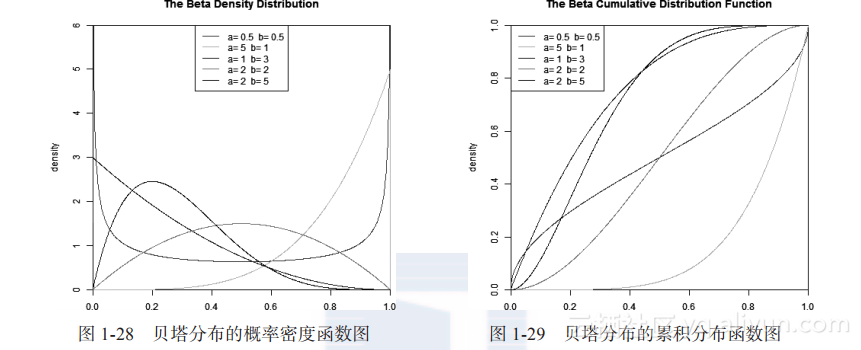3.?分布检验

set.seed(1)
S<-rbeta(1000,1,2)
ks.test(S, "pbeta",1,2) # Kolmogorov-Smirnov检验

One-sample Kolmogorov-Smirnov test

data: S

D = 0.0202, p-value = 0.807
alternative hypothesis: two-sided